# Face Detection

In this tutorial, we’ll see how to create and launch a face detection algorithm in Python using OpenCV. We’ll also add some features to detect eyes and mouth on multiple faces at the same time. This article will go through the most basic implementations of face detection including Cascade Classifiers, HOG windows and Deep Learning.

We’ll cover face detection using :

• Haar Cascade Classifiers using OpenCV
• Histogram of Oriented Gradients using Dlib
• Convolutional Neural Networks using Dlib

# Introduction

We’ll be using OpenCV, an open source library for computer vision, written in C/C++, that has interfaces in C++, Python and Java. It supports Windows, Linux, MacOS, iOS and Android. Some of our work will also require using Dlib, a modern C++ toolkit containing machine learning algorithms and tools for creating complex software.

## Requirements

The first step is to install OpenCV. Run the following command line in your terminal :

pip install opencv-python


Depending on your version, the file will be installed here :

/usr/local/lib/python3.7/site-packages/cv2


If you have not yet installed Dlib, run the following command :

pip install dlib


## Imports and models path

We’ll create a new Jupyter notebook / python file and start off with :

import cv2
import matplotlib.pyplot as plt
import dlib
from imutils import face_utils

font = cv2.FONT_HERSHEY_SIMPLEX


We’ll explore Cascade Classifiers at first.

## 1. Theory

Cascade classifier, or namely cascade of boosted classifiers working with haar-like features, is a special case of ensemble learning, called boosting. It typically relies on Adaboost classifiers (and other models such as Real Adaboost, Gentle Adaboost or Logitboost).

Cascade classifiers are trained on a few hundred sample images of image that contain the object we want to detect, and other images that do not contain those images.

How can we detect if a face is there or not ? There is an algorithm, called Viola–Jones object detection framework, that includes all the steps required for live face detection :

• Haar Feature Selection, features derived from Haar wavelets
• Create integral image

The original paper was published in 2001.

### a. Haar Feature Selection

There are some common features that we find on most common human faces :

• a dark eye region compared to upper-cheeks
• a bright nose bridge region compared to the eyes
• some specific location of eyes, mouth, nose…

The characteristics are called Haar Features. The feature extraction process will look like this :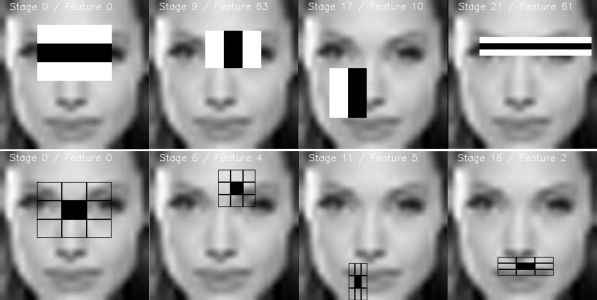In this example, the first feature measures the difference in intensity between the region of the eyes and a region across the upper cheeks. The feature value is simply computed by summing the pixels in the black area and subtracting the pixels in the white area.

$Rectangle Feature = \sum (pixels_{black area}) - \sum (pixels_{white area})$

Then, we apply this rectangle as a convolutional kernel, over our whole image. In order to be exhaustive, we should apply all possible dimensions and positions of each kernel. A simple 24*24 images would typically result in over 160’000 features, each made of a sum/subtraction of pixels values. It would computationally be impossible for live face detection. So, how do we speed up this process ?

• once the good region has been identified by a rectangle, it is useless to run the window over a completely different region of the image. This can be achieved by Adaboost.
• compute the rectangle features using the integral image principle, which is way faster. We’ll cover this in the next section.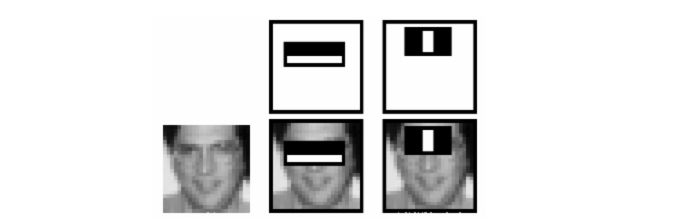There are several types of rectangles that can be applied for Haar Features extraction. According to the original paper :

• the two-rectangle feature is the difference between the sum of the pixels within two rectangular regions, used mainly for detecting edges (a,b)
• the three-rectangle feature computes the sum within two outside rectangles subtracted from the sum in a center rectangle, used mainly for detecting lines (c,d)
• the four-rectangle feature computes the difference between diagonal pairs of rectangle (e)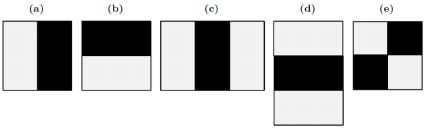Now that the features have been selected, we apply them on the set of training images using Adaboost classification, that combines a set of weak classifiers to create an accurate ensemble model. With 200 features (instead of 160’000 initially), an accuracy of 95% is acheived. The authors of the paper have selected 6’000 features.

### b. The integral image

Computing the rectangle features in a convolutional kernel style can be long, very long. For this reason, the authors, Viola and Jones, proposed an intermediate representation for the image : the integral image. The role of the integral image is to allow any rectangular sum to be computed simply, using only four values. We’ll see how it works !

Suppose we want to determine the rectangle features at a given pixel with coordinates $$(x,y)$$. Then, the integral image of the pixel in the sum of the pixels above and to the left of the given pixel.

$ii(x,y) = \sum_{x'≤x, y'≤y} i(x', y')$

where $$ii(x,y)$$ is the integral image and $$i(x,y)$$ is the original image.

When you compute the whole integral image, there is a form a recurrence which requires only one pass over the original image. Indeed, we can define the following pair of recurrences :

$s(x,y) = s(x,y-1) + i(x,y)$ $ii(x,y) = ii (x-1,y) + s(x,y)$

where $$s(x,y)$$ is the cumulative row sum and and $$s(x-1) = 0, ii(-1,y) = 0$$.

How can that be useful ? Well, consider a region D for which we would like to estimate the sum of the pixels. We have defined 3 other regions : A, B and C.

• The value of the integral image at point 1 is the sum of the pixels in rectangle A - The value at point 2 is A + B
• The value at point 3 is A + C
• The value at point 4 is A + B + C + D.

Therefore, the sum of pixels in region D can simply be computed as : $$4 + 1 - (2+3)$$.

And over a single pass, we have computed the value inside a rectangle using only 4 array references.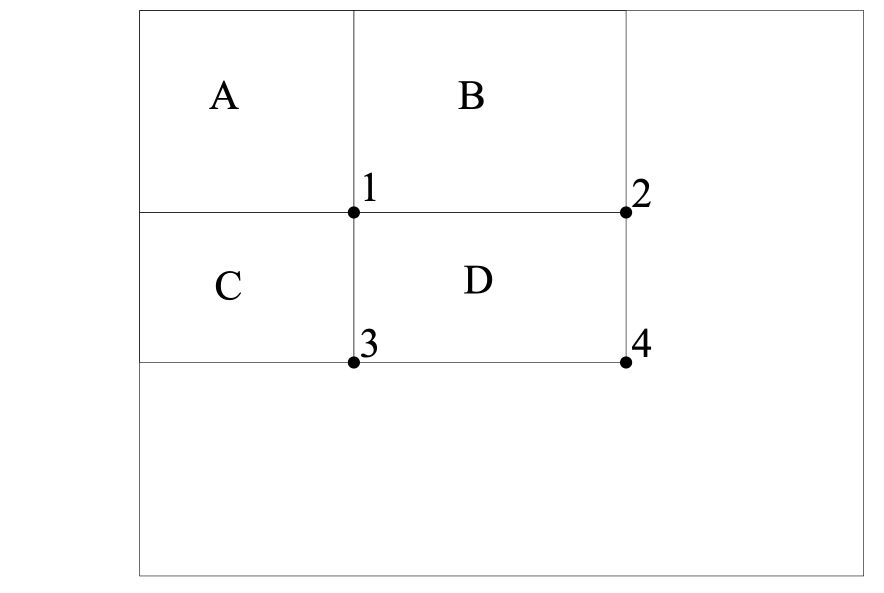One should simply be aware that rectangles are quite simple features in practice, but sufficient for face detection. Steerable filters tend to be more flexible when it comes to complex problems.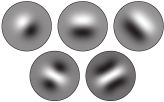### c. Learning the classification function with Adaboost

Given a set of labeled training images (positive or negative), Adaboost is used to :

• select a small set of features
• and train the classifier

Since most features among the 160’000 are supposed to be quite irrelevant, the weak learning algorithm around which we build a boosting model is designed to select the single rectangle feature which splits best negative and positive examples.

Although the process described above is quite efficient, a major issue remains. In an image, most of the image is a non-face region. Giving equal importance to each region of the image makes no sense, since we should mainly focus on the regions that are most likely to contain a picture. Viola and Jones achieved an increased detection rate while reducing computation time using Cascading Classifiers.

The key idea is to reject sub-windows that do not contain faces while identifying regions that do. Since the task is to identify properly the face, we want to minimize the false negative rate, i.e the sub-windows that contain a face and have not been identified as such.

A series of classifiers are applied to every sub-window. These classifiers are simple decision trees :

• if the first classifier is positive, we move on to the second
• if the second classifier is positive, we move on to the third

Any negative result at some point leads to a rejection of the sub-window as potentially containing a face. The initial classifier eliminates most negative examples at a low computational cost, and the following classifiers eliminate additional negative examples but require more computational effort.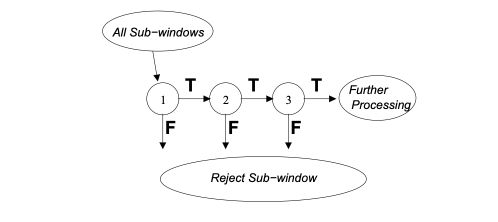The classifiers are trained using Adaboost and adjusting the threshold to minimize the false rate. When training such model, the variables are the following :

• the number of classifier stages
• the number of features in each stage
• the threshold of each stage

Luckily in OpenCV, this whole model is already pre-trained for face detection.

If you’d like to know more on Boosting techniques, I invite you to check my article on AdaBoost and Boosting.

## 2. Imports

The next step simply is to locate the pre-trained weights. We will be using default pre-trained models to detect face, eyes and mouth. Depending on your version of Python, the files should be located somewhere over here :

/usr/local/lib/python3.7/site-packages/cv2/data


Once identified, we’ll declare Cascade classifiers this way :

cascPath = "/usr/local/lib/python3.7/site-packages/cv2/data/haarcascade_frontalface_default.xml"



## 3. Detect face on an image

Before implementing the real time face detection algorithm, let’s try a simple version on an image. We can start by loading a test image :

# Load the image

plt.figure(figsize=(12,8))
plt.imshow(gray, cmap='gray')
plt.show()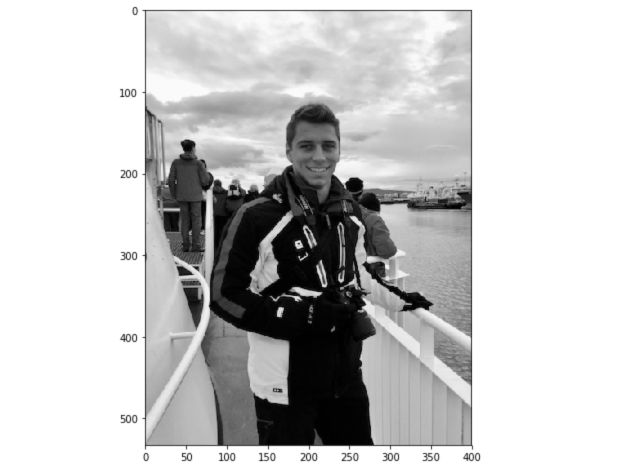Then, we detect the face and we add a rectangle around it :

# Detect faces
gray,
scaleFactor=1.1,
minNeighbors=5,
)

# For each face
for (x, y, w, h) in faces:
# Draw rectangle around the face
cv2.rectangle(gray, (x, y), (x+w, y+h), (255, 255, 255), 3)


Here is a list of the most common parameters of the detectMultiScale function :

• scaleFactor : Parameter specifying how much the image size is reduced at each image scale.
• minNeighbors : Parameter specifying how many neighbors each candidate rectangle should have to retain it.
• minSize : Minimum possible object size. Objects smaller than that are ignored.
• maxSize : Maximum possible object size. Objects larger than that are ignored.

Finally, display the result :

plt.figure(figsize=(12,8))
plt.imshow(gray, cmap='gray')
plt.show()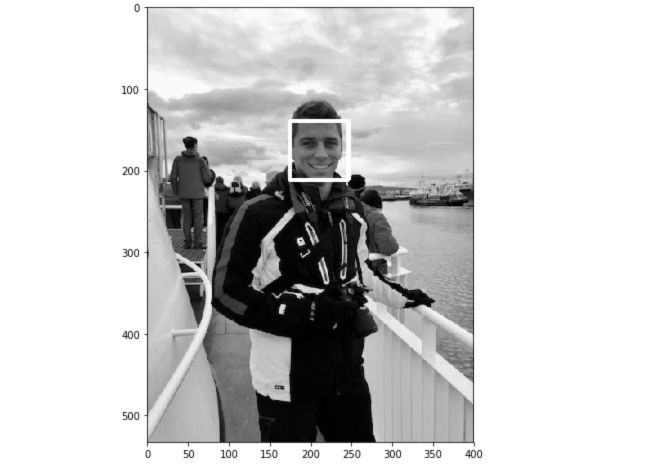Face detection works well on our test image. Let’s move on to real time now !

## 4. Real time face detection

Let’s move on to the Python implementation of the live facial detection. The first step is to launch the camera, and capture the video. Then, we’ll transform the image to a gray scale image. This is used to reduce the dimension of the input image. Indeed, instead of 3 points per pixel describing Red, Green, Blue, we apply a simple linear transformation :

$Y_{gray} = 0.2126R +0.7152G +0.0722B$

This is implemented by default in OpenCV.

video_capture = cv2.VideoCapture(0)

while True:
# Capture frame-by-frame
gray = cv2.cvtColor(frame, cv2.COLOR_BGR2GRAY)


Now, we’ll use the faceCascade variable define above, which contains a pre-trained algorithm, and apply it to the gray scale image.

    faces = faceCascade.detectMultiScale(
gray,
scaleFactor=1.1,
minNeighbors=5,
minSize=(30, 30),
)


For each face detected, we’ll draw a rectangle around the face :

    for (x, y, w, h) in faces:
if w > 250 :
cv2.rectangle(frame, (x, y), (x+w, y+h), (255, 0, 0), 3)
roi_gray = gray[y:y+h, x:x+w]
roi_color = frame[y:y+h, x:x+w]


For each mouth detected, draw a rectangle around it :

    smile = smileCascade.detectMultiScale(
roi_gray,
scaleFactor= 1.16,
minNeighbors=35,
minSize=(25, 25),
)
for (sx, sy, sw, sh) in smile:
cv2.rectangle(roi_color, (sh, sy), (sx+sw, sy+sh), (255, 0, 0), 2)
cv2.putText(frame,'Smile',(x + sx,y + sy), 1, 1, (0, 255, 0), 1)


For each eye detected, draw a rectangle around it :

    eyes = eyeCascade.detectMultiScale(roi_gray)
for (ex,ey,ew,eh) in eyes:
cv2.rectangle(roi_color,(ex,ey),(ex+ew,ey+eh),(0,255,0),2)
cv2.putText(frame,'Eye',(x + ex,y + ey), 1, 1, (0, 255, 0), 1)


Then, count the total number of faces, and display the overall image :

    cv2.putText(frame,'Number of Faces : ' + str(len(faces)),(40, 40), font, 1,(255,0,0),2)
# Display the resulting frame
cv2.imshow('Video', frame)


And implement an exit option when we want to stop the camera by pressing q :

    if cv2.waitKey(1) & 0xFF == ord('q'):
break


Finally, when everything is done, release the capture and destroy all windows. There are some troubles killing windows on Mac which might require killing Python from the Activity Manager later on.

video_capture.release()
cv2.destroyAllWindows()


## 5. Wrapping it up

import cv2

font = cv2.FONT_HERSHEY_SIMPLEX
video_capture = cv2.VideoCapture(0)

while True:
# Capture frame-by-frame

gray = cv2.cvtColor(frame, cv2.COLOR_BGR2GRAY)

gray,
scaleFactor=1.1,
minNeighbors=5,
minSize=(200, 200),
)

# Draw a rectangle around the faces
for (x, y, w, h) in faces:
cv2.rectangle(frame, (x, y), (x+w, y+h), (255, 0, 0), 3)
roi_gray = gray[y:y+h, x:x+w]
roi_color = frame[y:y+h, x:x+w]
cv2.putText(frame,'Face',(x, y), font, 2,(255,0,0),5)

roi_gray,
scaleFactor= 1.16,
minNeighbors=35,
minSize=(25, 25),
)

for (sx, sy, sw, sh) in smile:
cv2.rectangle(roi_color, (sh, sy), (sx+sw, sy+sh), (255, 0, 0), 2)
cv2.putText(frame,'Smile',(x + sx,y + sy), 1, 1, (0, 255, 0), 1)

for (ex,ey,ew,eh) in eyes:
cv2.rectangle(roi_color,(ex,ey),(ex+ew,ey+eh),(0,255,0),2)
cv2.putText(frame,'Eye',(x + ex,y + ey), 1, 1, (0, 255, 0), 1)

cv2.putText(frame,'Number of Faces : ' + str(len(faces)),(40, 40), font, 1,(255,0,0),2)
# Display the resulting frame
cv2.imshow('Video', frame)

if cv2.waitKey(1) & 0xFF == ord('q'):
break

# When everything is done, release the capture
video_capture.release()
cv2.destroyAllWindows()


# II. Histogram of Oriented Gradients (HOG) in Dlib

The second most popular implement for face detection is offered by Dlib and uses a concept called Histogram of Oriented Gradients (HOG). This is an implementation of the original paper by Dalal and Triggs.

## 1. Theory

The idea behind HOG is to extract features into a vector, and feed it into a classification algorithm like a Support Vector Machine for example that will assess whether a face (or any object you train it to recognize actually) is present in a region or not.

The features extracted are the distribution (histograms) of directions of gradients (oriented gradients) of the image. Gradients are typically large around edges and corners and allow us to detect those regions.

In the original paper, the process was implemented for human body detection, and the detection chain was the following :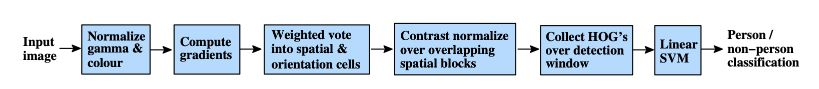### a. Preprocessing

First of all, the input images must but of the same size (crop and rescale images). The patches we’ll apply require an aspect ratio of 1:2, so the dimensions of the input images might be 64x128 or 100x200 for example.

### b. Compute the gradient images

The first step is to compute the horizontal and vertical gradients of the image, by applying the following kernels :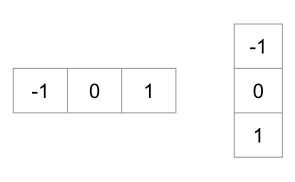The gradient of an image typically removes non-essential information.

The gradient of the image we were considering above can be found this way in Python :

gray = cv2.imread('images/face_detect_test.jpeg', 0)

im = np.float32(gray) / 255.0

gx = cv2.Sobel(im, cv2.CV_32F, 1, 0, ksize=1)
gy = cv2.Sobel(im, cv2.CV_32F, 0, 1, ksize=1)
mag, angle = cv2.cartToPolar(gx, gy, angleInDegrees=True)


And plot the picture :

plt.figure(figsize=(12,8))
plt.imshow(mag)
plt.show()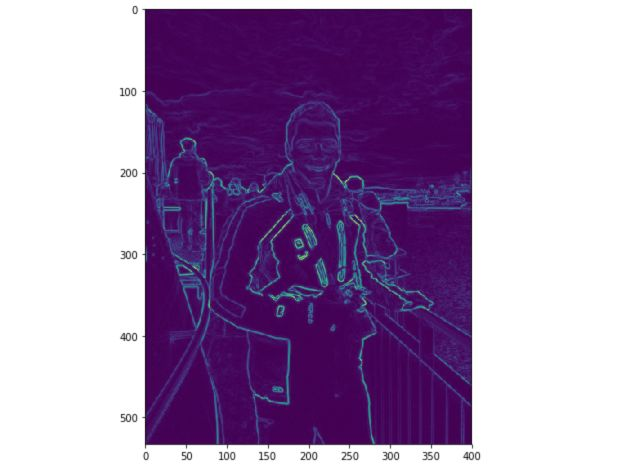We have not pre-processed the image before though.

### c. Compute the HOG

The image is then divided into 8x8 cells to offer a compact representation and make our HOG more robust to noise. Then, we compute a HOG for each of those cells.

To estimate the direction of a gradient inside a region, we simply build a histogram among the 64 values of the gradient directions (8x8) and their magnitude (another 64 values) inside each region. The categories of the histogram correspond to angles of the gradient, from 0 to 180°. Ther are 9 categories overall : 0°, 20°, 40°… 160°.

The code above gave us 2 information :

• and magnitude of the gradient

When we build the HOG, there are 3 subcases :

• the angle is smaller than 160° and not halfway between 2 classes. In such case, the angle will be added in the right category of the HOG
• the angle is smaller than 160° and exactly between 2 classes. In such case, we consider an equal contribution to the 2 nearest classes and split the magnitude in 2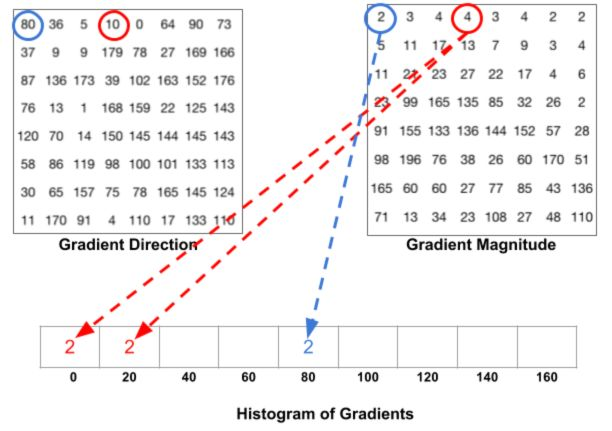• the angle is larger than 160°. In such case, we consider that the pixel contributed proportionally to 160° and to 0°.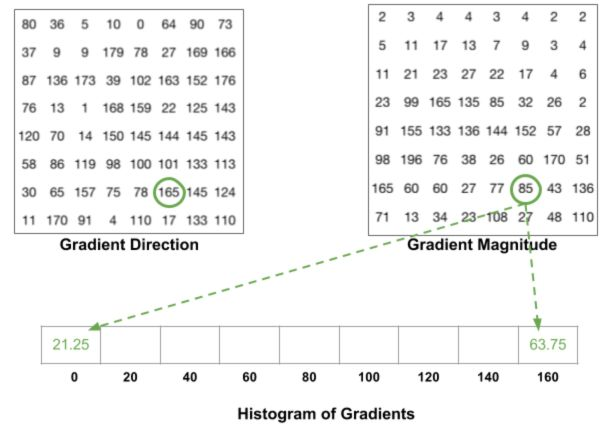The HOG looks like this for each 8x8 cell :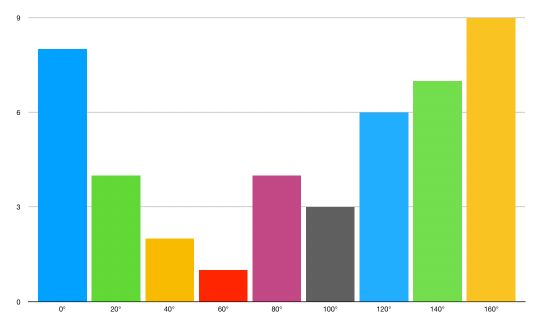### d. Block normalization

Finally, a 16x16 block can be applied in order to normalize the image and make it invariant to lighting for example. This is simply achieved by dividing each value of the HOG of size 8x8 by the L2-norm of the HOG of the 16x16 block that contains it, which is in fact a simple vector of length 9*4 = 36.

### e. Block normalization

Finally, all the 36x1 vectors are concatenated into a large vector. And we are done ! We have our feature vector, on which we can train a soft SVM classifier (C=0.01).

## 2. Detect face on an image

The implementation is pretty straight forward :

face_detect = dlib.get_frontal_face_detector()

rects = face_detect(gray, 1)

for (i, rect) in enumerate(rects):
(x, y, w, h) = face_utils.rect_to_bb(rect)
cv2.rectangle(gray, (x, y), (x + w, y + h), (255, 255, 255), 3)

plt.figure(figsize=(12,8))
plt.imshow(gray, cmap='gray')
plt.show()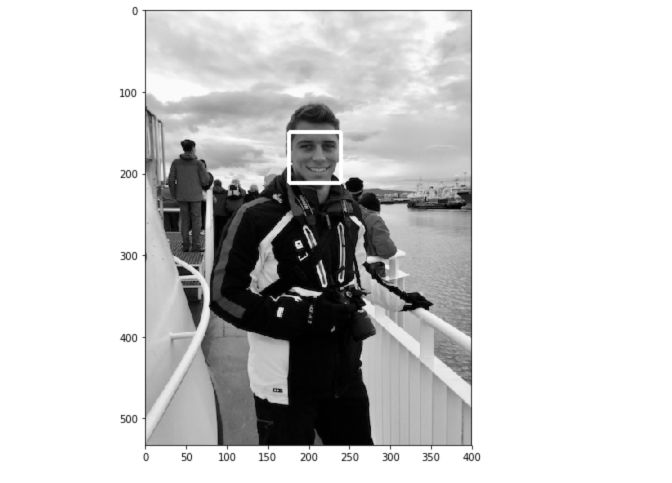## 3. Real time face detection

As previously, the algorithm is pretty easy to implement. We are also implementing a lighter version by detecting only the face. Dlib makes it really easy to detect facial keypoints too, but it’s another topic.

video_capture = cv2.VideoCapture(0)
flag = 0

while True:

gray = cv2.cvtColor(frame, cv2.COLOR_BGR2GRAY)
rects = face_detect(gray, 1)

for (i, rect) in enumerate(rects):

(x, y, w, h) = face_utils.rect_to_bb(rect)

cv2.rectangle(frame, (x, y), (x + w, y + h), (0, 255, 0), 2)

cv2.imshow('Video', frame)

if cv2.waitKey(1) & 0xFF == ord('q'):
break

video_capture.release()
cv2.destroyAllWindows()


# III. Convolutional Neural Network in Dlib

This last method is based on Convolutional Neural Networks (CNN). It also implements a paper on Max-Margin Object Detection (MMOD) for enhanced results.

## 1. A bit of theory

Convolutional Neural Network (CNN) are feed-forward neural network that are mostly used for computer vision. They offer an automated image pre-treatment as well as a dense neural network part. CNNs are special types of neural networks for processing datas with grid-like topology. The architecture of the CNN is inspired by the visual cortex of animals.

In previous approaches, a great part of the work was to select the filters in order to create the features in order to extract as much information from the image as possible. With the rise of deep learning and greater computation capacities, this work can now be automated. The name of the CNNs comes from the fact that we convolve the initial image input with a set of filters. The parameter to choose remains the number of filters to apply, and the dimension of the filters. The dimension of the filter is called the stride length. Typical values for the stride lie between 2 and 5.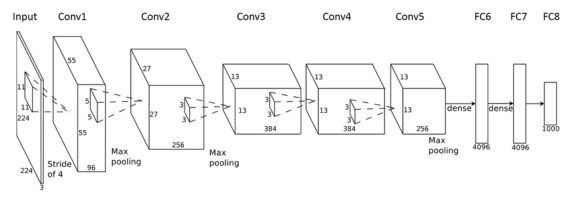The output of the CNN in this specific case is a binary classification, that takes value 1 if there is a face, 0 otherwise.

## 2. Detect face on an image

Some elements change in the implementation.

The first step is to download the pre-trained model here. Move the weights to your folder, and define dnnDaceDetector :

dnnFaceDetector = dlib.cnn_face_detection_model_v1("mmod_human_face_detector.dat")


Then, quite similarly to what we have done so far :

rects = dnnFaceDetector(gray, 1)

for (i, rect) in enumerate(rects):

x1 = rect.rect.left()
y1 = rect.rect.top()
x2 = rect.rect.right()
y2 = rect.rect.bottom()

# Rectangle around the face
cv2.rectangle(gray, (x1, y1), (x2, y2), (255, 255, 255), 3)

plt.figure(figsize=(12,8))
plt.imshow(gray, cmap='gray')
plt.show()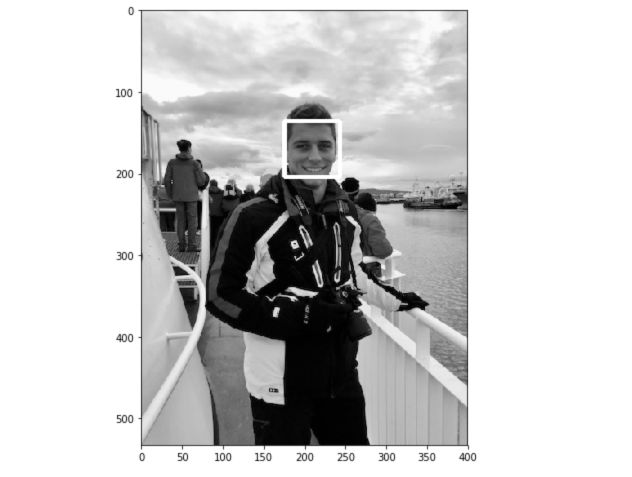## 3. Real time face detection

Finally, we’ll implement the real time version of the CNN face detection :

video_capture = cv2.VideoCapture(0)
flag = 0

while True:
# Capture frame-by-frame

gray = cv2.cvtColor(frame, cv2.COLOR_BGR2GRAY)
rects = dnnFaceDetector(gray, 1)

for (i, rect) in enumerate(rects):

x1 = rect.rect.left()
y1 = rect.rect.top()
x2 = rect.rect.right()
y2 = rect.rect.bottom()

# Rectangle around the face
cv2.rectangle(frame, (x1, y1), (x2, y2), (0, 255, 0), 2)

# Display the video output
cv2.imshow('Video', frame)

# Quit video by typing Q
if cv2.waitKey(1) & 0xFF == ord('q'):
break

video_capture.release()
cv2.destroyAllWindows()


# 4. Which one to choose ?

Tough question, but we’ll just go through 2 metrics that are important :

• the computation time
• the accuracy

In terms of speed, HoG seems to be the fastest algorithm, followed by Haar Cascade classifier and CNNs.

However, CNNs in Dlib tend to be the most accurate algorithm. HoG perform pretty well but have some issues identifying small faces. HaarCascade Classifiers perform around as good as HoG overall.

I have personally used mainly HoG in my personal projects due to its speed for live face detection.